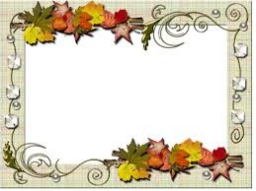# Photos

Three friends, Mirka, Lucka, and Hanka, collect photos. If Mirka had three more photos, she would have as many as Hanka. If Lucka had five photos less than she has, she would be like Hanka. How many photographs does each have if they have 431 together?

H =  143
L =  148
M =  140

### Step-by-step explanation:

M+L+H = 431
M+3 = H
L-5 = H

H+L+M = 431
H-M = 3
H-L = -5

Row 2 - Row 1 → Row 2
H+L+M = 431
-L-2M = -428
H-L = -5

Row 3 - Row 1 → Row 3
H+L+M = 431
-L-2M = -428
-2L-M = -436

Pivot: Row 2 ↔ Row 3
H+L+M = 431
-2L-M = -436
-L-2M = -428

Row 3 - -1/-2 · Row 2 → Row 3
H+L+M = 431
-2L-M = -436
-1.5M = -210

M = -210/-1.5 = 140
L = -436+M/-2 = -436+140/-2 = 148
H = 431-L-M = 431-148-140 = 143

H = 143
L = 148
M = 140

Our linear equations calculator calculates it.Did you find an error or inaccuracy? Feel free to write us. Thank you!

Tips for related online calculators
Do you have a system of equations and looking for calculator system of linear equations?